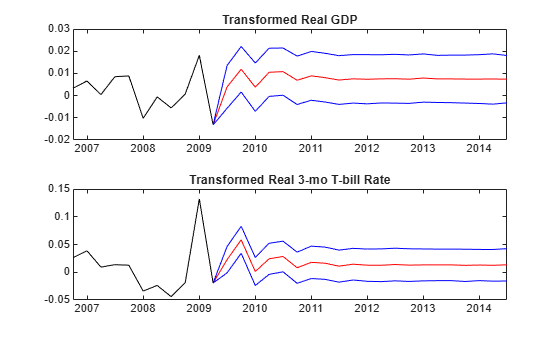# Forecast VAR Model Using Monte Carlo Simulation

This example shows how to use Monte Carlo simulation via `simulate` to forecast a VAR model.

`simulate` enables you to generate simulations of time series based on your model. If you have a trustworthy VAR model object, you can use these simulations as sample forecasts.

`simulate` requires:

• A model (`EstMdl` in what follows)

• The number of periods for the forecast (`numobs` in what follows)

`simulate` optionally takes:

• An exogenous data series

• A presample time series (`Y(end-3:end,:)` in what follows)

• Future sample responses for conditional simulation

• The number of realizations, or paths, to simulate (`2000` in what follows)

Load the `Data_USEconModel` data set. This example uses two time series: the logarithm of real GDP, and the real 3-month T-bill rate, both differenced to be approximately stationary. For illustration, a VAR(4) model describes the time series.

```load Data_USEconModel DEF = log(DataTimeTable.CPIAUCSL); GDP = log(DataTimeTable.GDP); rGDP = diff(GDP - DEF); % Real GDP is GDP - deflation TB3 = 0.01*DataTimeTable.TB3MS; dDEF = 4*diff(DEF); % Scaling rTB3 = TB3(2:end) - dDEF; % Real interest is deflated Y = [rGDP,rTB3];```

Fit a VAR(4) model specification.

```Mdl = varm(2,4); Mdl.SeriesNames = {'Transformed real GDP','Transformed real 3-mo T-bill rate'}; EstMdl = estimate(Mdl,Y);```

Define the forecast horizon.

```numobs = 21; FDates = dateshift(DataTimeTable.Time(end),'end','quarter',1:numobs);```

Simulate the model for `numobs` steps ahead, and generate 2000 paths. Specify presample observations from the end of the data.

```rng(1); %For reproducibility Ysim = simulate(EstMdl,numobs,'Y0',Y(end-3:end,:),'NumPaths',2000);```

Calculate the mean and standard deviation of the simulated series:

```Ymean = mean(Ysim,3); % Calculate means Ystd = std(Ysim,0,3); % Calculate std deviations```

Plot the means +/- 1 standard deviation for the simulated series:

```figure; subplot(2,1,1) plot(DataTimeTable.Time(end-10:end),Y(end-10:end,1),'k') hold('on') plot([DataTimeTable.Time(end) FDates],[Y(end,1);Ymean(:,1)],'r') plot([DataTimeTable.Time(end) FDates],[Y(end,1);Ymean(:,1)]+[0;Ystd(:,1)],'b') plot([DataTimeTable.Time(end) FDates],[Y(end,1);Ymean(:,1)]-[0;Ystd(:,1)],'b') title('Transformed Real GDP') subplot(2,1,2) plot(DataTimeTable.Time(end-10:end),Y(end-10:end,2),'k') hold('on') plot([DataTimeTable.Time(end) FDates],[Y(end,2);Ymean(:,2)],'r') plot([DataTimeTable.Time(end) FDates],[Y(end,2);Ymean(:,2)]+[0;Ystd(:,2)],'b') plot([DataTimeTable.Time(end) FDates],[Y(end,2);Ymean(:,2)]-[0;Ystd(:,2)],'b') title('Transformed Real 3-mo T-bill Rate')```